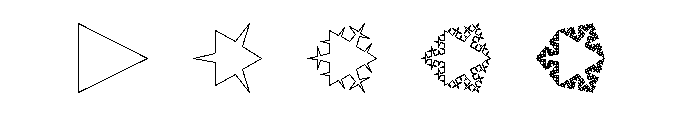MAT331 - Project 3

Fractals

This project is concerned with ``fractals'', that is, objects whose Hausdorff dimension is greater than their topological dimension (or, roughly, ``things which are so wiggly we have to say their dimension is not an integer''). Notice that I am purposfully omitting a precise definition here; first, that's your job, and second, there isn't a single definition that everyone accepts. If you like, you may stick to self-similar fractals, as we have in class.

As usual, this project has both an expository and a computational portion. For the expository part, you should write a short paper (3-5 pages or so) on fractals, dimension, etc. You will probably want to refer to books and articles in the library(good possibilities are books by Peitgen/Jürgens/Saupe; Falconer; or chapter 4 of the "Chaos" text by Alligood/Sauer/Yorke). You might also want to search the World-Wide-Web; there is quite a lot of information on fractals on the web, but be careful: as is always true, some of the information on the web is misguided, misleading, or just downright wrong.

For the computational part, you should make some fractals listed below. You may use the "turtle" approch as we discussed in class, or write programs to make them directly, as you prefer. I would suggest that you incorporate the fractals you compute in the computational portion into the expository portion, using them as illustrations, etc. Then include the maple to do them as an appendix.

1. Make a vonKoch snowflake, then modify it so that it becomes "spikier" or "flatter" (see pictures below). Compute the dimension for each case.2 . Consider the fractal made by taking a square, then repeatedly replacing each side by 8 segments each 1/4 as long, arranged as below. What is the resulting figure? Compute its dimension.What if you replace each segment of a square by 9 segments each 1/3 as long, arranged as below? What is the resulting figure, and what is its dimension?3 . Make the following fractal. Compute its dimension.4 . (optional for extra credit). Create one or more other fractals. Discuss, guess at, or compute their dimensions. The amount of extra credit will be based on originality, creativity, and correctness.